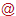Leibniz Institute for Plasma Science and Technology
Felix-Hausdorff-Str. 2
17489 Greifswald
GERMANY

https://www.inp-greifswald.de/en/
welcomeinp-greifswald [punkt] de

The Leibniz Institute for Plasma Science and Technology (INP) is the largest non-university institute in the field of low temperature plasmas, their basics and technical applications in Europe. The institute carries out research and development from idea to prototype. The topics focus on the needs of the market. At present, plasmas for materials and energy as well as for environment and health are the focus of interest.

## Electrical characteristics of atmospheric-pressure DBD in argon with small admixtures of TMS - measured and calculated data

A time-dependent, spatially one-dimensional fluid-Poisson model has been applied to analyse the impact of small amounts of tetramethylsilane (TMS) on the discharge characteristics of an atmospheric-pressure dielectric barrier discharge (DBD) in argon. Based on an established argon kinetics, it includes a reaction kinetics for TMS, which has been validated by measurements of the ignition voltage at the frequency f = 86.2 kHz for TMS amounts of up to 200 ppm. It is found that good agreement between measured and calculated ignition voltage can be obtained, when assuming that 25 % of the reactions of TMS with excited argon atoms with a rate coefficient of 3.0×10^{−16} m^3/s lead to the production of electrons due to Penning ionization. The dataset contains measured and calculated data for the ignition voltage and discharge current.

FieldValue
Group
Authors
Release Date
2020-08-12
Identifier
747f7a1e-861e-4329-a1bb-2695a58b3acb
Permanent Identifier (DOI)
Permanent Identifier (URI)
Is supplementing
Plasma Source Name
Plasma Source Application
Plasma Source Specification
Plasma Source Properties

Atmospheric-pressure DBD between plane steel mesh electrodes covered by dielectrics (borofloat glass, relative permittivity: 4.6); Electrode area: 8 cm^2; Thickness of dielectrics: 2 mm; Discharge gap: 1 mm; Voltage supply: sinusoidal voltage with variable amplitude and frequency of 86.2 kHz

Plasma Medium Name
Plasma Medium Properties

Gas temperature: 300 K; Pressure: 1 atm; Gas mixture: Ar + 0 to 200 ppm TMS

Plasma Diagnostics Name
Plasma Diagnostics Properties

Fluid-Poisson model:
Mesh size: Non-equidistant spatial grid using typically 250 intervals with logarithmically refined mesh towards the boundaries; Time step size: adaptive time-step control

Voltage measurement:
HV probe: PHV 4002-3, dataTec, Reutlingen, Germany; Oscilloscope: MDO3052, Tektronix, Beaverton, OR, USA

Current measurement:
Oscilloscope: MDO3052 from Tektronix, Beaverton, OR, USA; Methods: shunt resistor method using 1.00 Ω precision resistor and monitor capacitor method using 32.5 nF measuring capacitor

Plasma Diagnostics Procedure

Fluid-Poisson model:
The time-dependent, spatially one-dimensional fluid-Poisson model includes particle balance equations for the densities of electrons, relevant neutral particles and ions, the electron energy balance equation to determine the mean electron energy, the Poisson equation to calculate the electric potential and field, as well as a balance equation for the surface charge density to consider the accumulation of charge carriers on the dielectric surfaces.

Voltage measurement:
To determine the ignition voltage U_i, the amplitude U_{a,0} of the applied voltage was increased step
by step for a specific Ar-TMS mixture until the discharge was ignited and covered the entire electrode area, i.e., U_i=U_{a,0}.

Current measurement:
The current I(t) was obtained by monitoring the voltage drop at the precision resistor or the transferred charge at the measuring capacitor.

Language
English